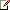# 静态类和静态类成员（C# 编程指南）

``````UtilityClass.MethodA();
``````

``````double dub = -3.14;
Console.WriteLine(Math.Abs(dub));
Console.WriteLine(Math.Floor(dub));
Console.WriteLine(Math.Round(Math.Abs(dub)));

// Output:
// 3.14
// -4
// 3
``````注意

• 仅包含静态成员。

• 无法实例化。

• 是密封的。

• 不能包含实例构造函数

``````public static class TemperatureConverter
{
public static double CelsiusToFahrenheit(string temperatureCelsius)
{
// Convert argument to double for calculations.
double celsius = Double.Parse(temperatureCelsius);

// Convert Celsius to Fahrenheit.
double fahrenheit = (celsius * 9 / 5) + 32;

return fahrenheit;
}

public static double FahrenheitToCelsius(string temperatureFahrenheit)
{
// Convert argument to double for calculations.
double fahrenheit = Double.Parse(temperatureFahrenheit);

// Convert Fahrenheit to Celsius.
double celsius = (fahrenheit - 32) * 5 / 9;

return celsius;
}
}

class TestTemperatureConverter
{
static void Main()
{
Console.WriteLine("1\. From Celsius to Fahrenheit.");
Console.WriteLine("2\. From Fahrenheit to Celsius.");
Console.Write(":");

double F, C = 0;

switch (selection)
{
case "1":
Console.Write("Please enter the Celsius temperature: ");
Console.WriteLine("Temperature in Fahrenheit: {0:F2}", F);
break;

case "2":
Console.Write("Please enter the Fahrenheit temperature: ");
Console.WriteLine("Temperature in Celsius: {0:F2}", C);
break;

default:
break;
}

// Keep the console window open in debug mode.
Console.WriteLine("Press any key to exit.");
}
}
/* Example Output:
1\. From Celsius to Fahrenheit.
2\. From Fahrenheit to Celsius.
:2
Please enter the Fahrenheit temperature: 20
Temperature in Celsius: -6.67
Press any key to exit.
*/
``````

## 静态成员

C# 不支持静态局部变量（在方法范围内声明的变量）。

``````public class Automobile
{
public static int NumberOfWheels = 4;
public static int SizeOfGasTank
{
get
{
return 15;
}
}
public static void Drive() { }
public static event EventType RunOutOfGas;

// Other non-static fields and properties...
}
``````

``````Automobile.Drive();
int i = Automobile.NumberOfWheels;
``````

C# 编程指南

static（C# 参考）

class（C# 参考）